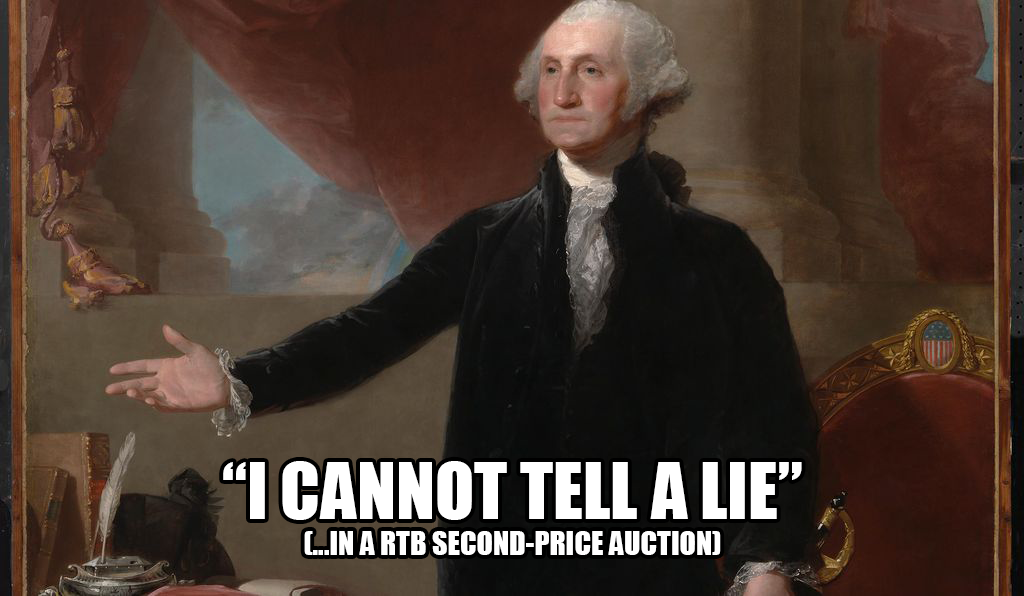# Proving the Mysterious Vickrey AuctionImage taken from google. Vickrey Auction can be extended to Real Time Bidding (RTB)

Vickrey auction is known for its “too good to be true” property. This type of auctions is designed in such a way that bidders will bid at their true valuations. In other words, they will not lie! How does that even work? Without further a do, let us use a simple game theory to prove that.

## The simple game theoretic proof¹

Consider the a hypothetical bidder that we call Albert. Albert has a valuation of v and a bid b. Remember that in Vickrey auction, each bidder will have to submit a sealed bid of their valuations in a closed envelope (or anything that is sealed). Let the highest bid among other bidders be B. In this situation, Albert will win the item if his bid of b is higher than B and he will pay a value of B for the item.

One technique in mathematics that we use to prove let say x = y value is by checking the boundary conditions. As such, to prove that Albert will bid at b = v, we need to check the boundary conditions. There are two boundary conditions here. One where c = b > v and the other where c = b < v.

## c = b > v

In this simple set up, there are three possible scenarios:

1. B > c > v
2. c > B > v
3. c > v > B

In scenario number 1, Albert will not win the bid. Since his bid of cis lower than the highest bid among other people, another person will win the bid. Changing c to v does not help either. He will always lose. As such, he might as well bid v from the very start. In scenario number 2, his bid of cis higher than B. As such, he will win the item and pays a total amount of money that is equal to B. However, B is higher than his true valuation of v. Therefore even though he wins the item, he will incur a loss of B - v! In this scenario, it is better to bid at v and lose on purpose! In scenario number 3, the same thing happens. Albert will win the item and pays B. His gain will be v - B because he only values the item as much as v. But in this case, he might as well bid v instead of c in the first place because the end gain is the same! Therefore when c = b > v, he has the incentive to bid v.

## c = b < v

In this simple set up, there are three possible scenarios:

1. v > c > B
2. v > B > c
3. B > v > c

In scenario number 1, Albert will win the item and pays B for it. In this case, his surplus or gain will be v - B. As such, he might as well bid v instead of c in the first place because it will yield the same gain anyway. In scenario number 2, Albert will lose the item. However if he bids v instead of c, he will turn from a loser to a winner! He could have gotten the item. So, he should have bid with v in the first place. In scenario number 3, Albert will lose the item regardless. As such, he might as well go with v. Therefore when c = b < v, under all possible scenarios Albert has the incentive to bid v instead of some other value.

## Q.E.D

 The proof can be found on wikipedia page. I also used my university notes to simply it.

--

--